Next: The SPC water Up: Theory Previous: The Lennard--Jones potential

## The Coulomb potential

The Lennard--Jones potential is one important part of the interaction between water molecules. The second part of the interaction in our Wasser model is due to forces between electrical charges. Although the water molecule is electrically neutral, the positive and negative charges it contains are not uniformly distributed, as will be described below. The result is that in addition to the induced dipole moment important in the Lennard--Jones potential, the water molecule has a permanent dipole moment, so that parts of adjacent water molecules attract and repel one another.

Interactions between water molecules due to their permanent dipole moments are described approximately by treating the charged portions of the molecule as point charges. Then we use the Coulomb potential for point charges to estimate the forces between the charged portions of each water molecule and the charged parts of its neighbors. The Coulomb potential is an effective pair potential that describes the interaction between two point charges. It acts along the line connecting the two charges. It is given by the equation: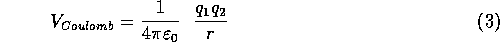where r is the distance between two ions,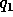and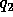the electric charge in coulombs carried by charge 1 and 2 respectively, and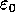is the electrical permittivity of space. Two oppositely charged particles will give an attractive potential, whereas if both particles are of the same sign (i.e., both are positive or both are negative) the potential is repulsive. The resulting potential is shown in Figure 3.6.1. For charges on water (partial charges on the hydrogen and oxygen atoms),andare comparable in magnitude to the elementary charge (the smallest conventional charge)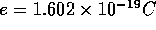.Fig. 3.6.1 The Coulomb potential. The bottom curve represents the interaction between two particles of opposite charge, the top curve represents the interaction of particles of equal charge.

The Coulomb force between the two molecules is: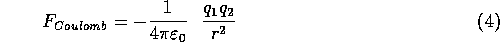Next: The SPC water Up: Theory Previous: The Lennard--Jones potential